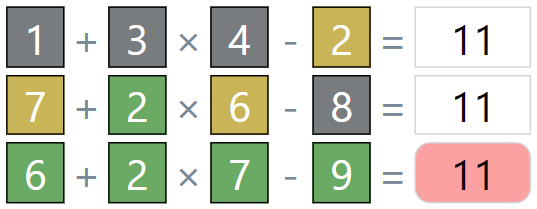Results copied to clipboardA puzzle to find where the numbers are placed

Use the numbers 1-9 once only - or 1-12
Click the red answer box to check the solution
Green = Correct number in correct position
Yellow = Number in wrong position
Grey = Number not present in equation
You have a maximum of six attempts
Daily Ooodle (1-12) result can be shared/tweeted

Try the Times Square Grid speed challengeRange:
Numbers 1 to 9

Timer

 5 x 5 + 5 - 5 = 5 x 5 + 5 - 5 = 5 x 5 + 5 - 5 = 5 x 5 + 5 - 5 = 5 x 5 + 5 - 5 = 5 x 5 + 5 - 5 =

Played
Win %
Current Streak
Max Streak

1
2
3
4
5
6